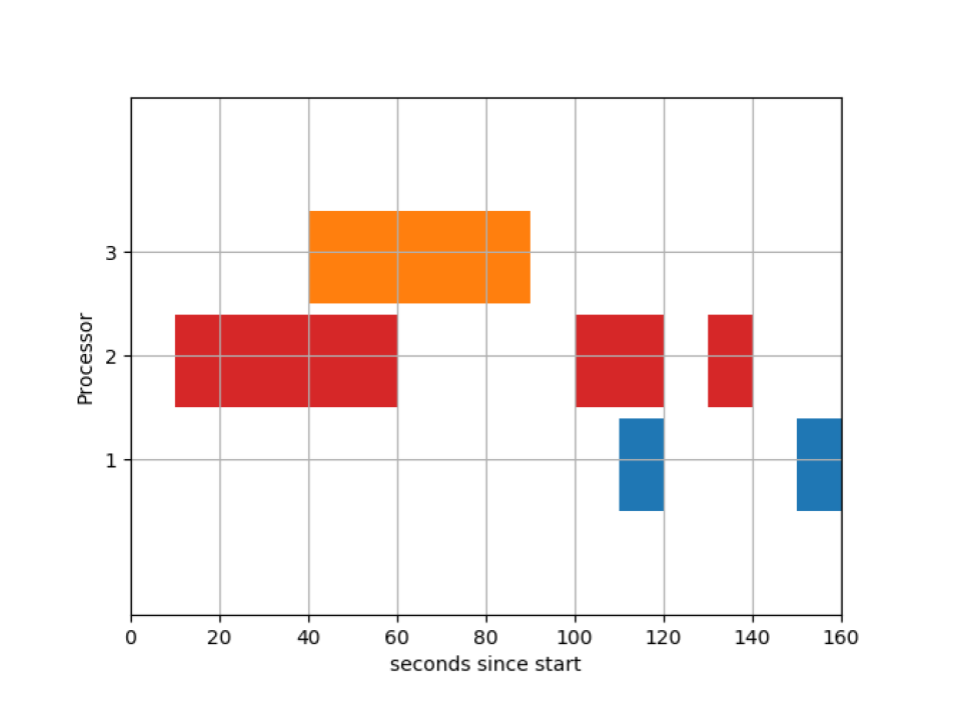# Python | Basic Gantt chart using Matplotlib

Prerequisites : Matplotlib Introduction
In this article, we will be discussing how to plot a Gantt Chart in Python using Matplotlib.
A Gantt chart is a graphical depiction of a project schedule or task schedule (In OS). It’s is a type of bar chart that shows the start and finish dates of several elements of a project that include resources or deadline. The first Gantt chart was devised in the mid-1890s by Karol Adamiecki, a Polish engineer who ran a steelworks in southern Poland and had become interested in management ideas and techniques. Some 15 years after Adamiecki, Henry Gantt, an American engineer, and project management consultant, devised his own version of the chart which became famous as “Gantt Charts”.
Some Uses of Gantt Charts :

• Project Scheduling.

A sample Gantt Chart for task scheduling is shown below:We will be using broken_barh types of graph available in matplotlib to draw gantt charts.
Below is the code for generating the above gantt chart :

## Python3

 `# Importing the matplotlib.pyplot` `import` `matplotlib.pyplot as plt`   `# Declaring a figure "gnt"` `fig, gnt ``=` `plt.subplots()`   `# Setting Y-axis limits` `gnt.set_ylim(``0``, ``50``)`   `# Setting X-axis limits` `gnt.set_xlim(``0``, ``160``)`   `# Setting labels for x-axis and y-axis` `gnt.set_xlabel(``'seconds since start'``)` `gnt.set_ylabel(``'Processor'``)`   `# Setting ticks on y-axis` `gnt.set_yticks([``15``, ``25``, ``35``])` `# Labelling tickes of y-axis` `gnt.set_yticklabels([``'1'``, ``'2'``, ``'3'``])`   `# Setting graph attribute` `gnt.grid(``True``)`   `# Declaring a bar in schedule` `gnt.broken_barh([(``40``, ``50``)], (``30``, ``9``), facecolors ``=``(``'tab:orange'``))`   `# Declaring multiple bars in at same level and same width` `gnt.broken_barh([(``110``, ``10``), (``150``, ``10``)], (``10``, ``9``),` `                         ``facecolors ``=``'tab:blue'``)`   `gnt.broken_barh([(``10``, ``50``), (``100``, ``20``), (``130``, ``10``)], (``20``, ``9``),` `                                  ``facecolors ``=``(``'tab:red'``))`   `plt.savefig(``"gantt1.png"``)`

Lets’s understand the different pieces of codes one by one :

`fig, gnt = plt.subplots()`
• Here, we declared a figure “gnt” for plotting the chart.
```gnt.set_ylim(0, 50)
gnt.set_xlim(0, 160)```
• Here, we declared the limits of X-axis and Y-axis of the chart. By default the lower X-axis and Y-axis limit is 0 and higher limits for both axis is 5 unit more the highest X-axis value and Y-axis value.
```gnt.set_xlabel('seconds since start')
gnt.set_ylabel('Processor')```
• Here, we added labels to the axes. By default, there is no labels.
```gnt.set_yticks([15, 25, 35])
gnt.set_yticklabels(['1', '2', '3'])```
• Here, we added ticks in Y-axis. We can also label them. By default the axes is divides uniformly in the limits.
`gnt.grid(True)`
• Here, we set grid() to True to show grids. By default, it is False.
`gnt.broken_barh([(40, 50)], (30, 9), facecolors=('tab:orange'))`
• Here, we added a bar in the chart. In this example, this bar represent the operation going on for time 40 to (40+50)= 90 sec.
The basic arguments :
```gnt.broken_barh([(start_time, duration)],
(lower_yaxis, height),
facecolors=('tab:colours'))```
• By default color is set to Blue.
We can declare multiple bars at the same time :
```gnt.broken_barh([(10, 50), (100, 20), (130, 10)], (20, 9),
facecolors=('tab:red'))```
• We can also add edge color by setting “edgecolor” attribute to any color.
`plt.savefig("gantt1.png")`
• We saved the figure formed in the png file.

Reference : Broken Barh Example Matplotlib Documentation

Whether you're preparing for your first job interview or aiming to upskill in this ever-evolving tech landscape, GeeksforGeeks Courses are your key to success. We provide top-quality content at affordable prices, all geared towards accelerating your growth in a time-bound manner. Join the millions we've already empowered, and we're here to do the same for you. Don't miss out - check it out now!

Previous
Next